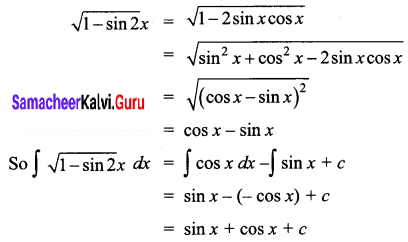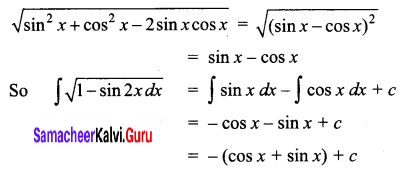# Samacheer Kalvi 12th Business Maths Solutions Chapter 2 Integral Calculus I Ex 2.4

Students can download 12th Business Maths Chapter 2 Integral Calculus I Ex 2.4 Questions and Answers, Samacheer Kalvi 12th Business Maths Book Solutions Guide Pdf helps you to revise the complete Tamilnadu State Board New Syllabus and score more marks in your examinations.

## Tamilnadu Samacheer Kalvi 12th Business Maths Solutions Chapter 2 Integral Calculus I Ex 2.4

Integrate the following with respect to x.

Question 1.
2 cos x – 3 sin x + 4 sec2 x – 5 cosec2 x
Solution:
∫2 cos x – 3 sin x + 4 sec2 x – 5 cosec2 x
= 2 ∫ cos x dx – 3 ∫ sin x dx + 4 ∫ sec2 x – 5 ∫ cosec2 x dx
= 2 sin x + 3 cos x + 4 tan x + 5 cot x + c

Question 2.
∫ sin3 x dx
Solution:
We know that, sin 3x = 3 sin x – 4 sin3 x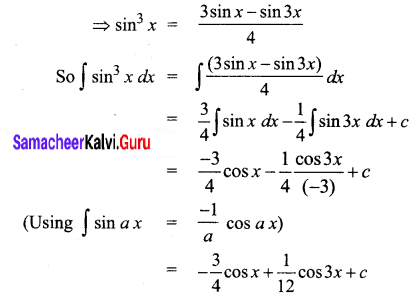Question 3.
$$\frac{\cos 2 x+2 \sin ^{2} x}{\cos ^{2} x}$$
Solution: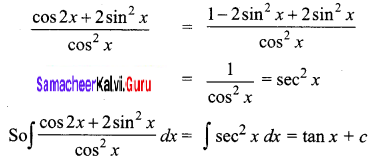Question 4.
$$\frac{1}{\sin ^{2} x \cos ^{2} x}$$
[Hint: sin2 x + cos2 x = 1]
Solution: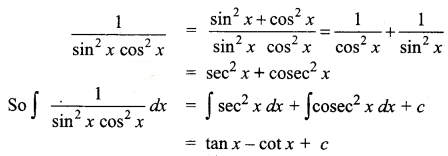Question 5.
$$\sqrt{1-\sin 2} x$$
Solution: